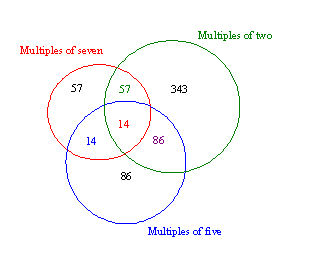#### You may also likeMake a set of numbers that use all the digits from 1 to 9, once and once only. Add them up. The result is divisible by 9. Add each of the digits in the new number. What is their sum? Now try some other possibilities for yourself!### Have You Got It?

Can you explain the strategy for winning this game with any target?### Counting Factors

Is there an efficient way to work out how many factors a large number has?

# Divisively So

##### Age 11 to 14 Challenge Level:

Matthew Turner (Queen Mary's Grammar School) sent us a lovely solution to this problem.

You could work with 999 as well as 1000 as the question says "numbers less than 1000" but the solution would have been the same (why?).

Here is Matthew's solution:By dividing 1000 by two I know that there are 500 multiples of two. By dividing 100 by five I know there are 200 multiples of five. By dividing by seven I know there are 142 multiples of seven.

To work out the how many multiples of five, two and seven, we have to find the LCM of them, which is 70. This goes into 1000 fourteen times, so 14 goes into the area where all three circles cross.

There are 100 numbers that are multiples of two and five (LCM 10) less the 14 that are also multiples of 7, leaves 86.

There are 71 numbers that are multiples of two and seven (LCM 14) less the 14 that are also multiples of 5, leaves 57.

There are 28 numbers that are multiples of seven and five (LCM 35) less the 14 that are also multiples of 2, leaves 14.

To work out how much was in the biggest parts of the circles I added up the other three parts and subtracted each of these figures from the total number of multiples of each of the numbers.

e.g. for two

57 + 86 + 14 = 157

500 - 157 = 343

Then we add up every number within the three circles which adds up to 657 and take that from 1000, which is 343.

For the answer to the first part we only need to consider the multiples of 2 and 5 so

343 + 57 + 86 + 14 + 86 + 14 = 600

1000 - 600 is 400.